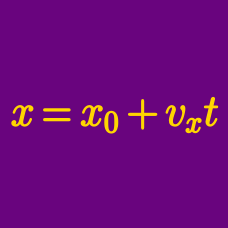Classical Mechanics

# Projectile motion - beginners

A $9 \text{ kg}$ body is projected horizontally at $12 \text{ m/s}$ from a height of $245 \text{ m}.$ What is the magnitude of the body's vertical velocity when it strikes the ground?

The air resistance is negligible and the gravitational acceleration is $g = 10 \text{ m/s}^2.$

A ball of mass $3 \text{ kg}$ is projected from the ground with a velocity of $30 \text{ m/s}$ in a direction that makes a $30 ^\circ$ angle with the horizon. How far does the ball fly horizontally?

Ignore any air resistance and assume that gravitational acceleration is $g = 10 \text{ m/s}^2.$

A body of mass $2 \text{ kg}$ is projected horizontally with a velocity of $3 \text{ m/s}$ from a height of $45 \text{ m}.$ How far does the body fly horizontally?

Ignore any air resistance and assume that gravitational acceleration is $g = 10 \text{ m/s}^2.$

A body of mass $6 \text{ kg}$ is projected horizontally with a velocity of $12 \text{ m/s}$ from a height of $80 \text{ m}.$ How long does it take for the body to touch the ground?

The air resistance is negligible and the gravitational acceleration is $g = 10 \text{ m/s}^2.$

A body of mass $6 \text{ kg}$ is projected horizontally with a velocity of $45 \text{ m/s}$ from a height of $180 \text{ m}.$ Find the magnitude of its velocity at the moment it touches the ground.

Ignore any air resistance and assume that gravitational acceleration is $g = 10 \text{ m/s}^2.$

×